### Generalized Maximum Entropy

Reparameterization of the errors in a regression equation is the process of specifying a support for the errors, observation by observation. If a two-point support is used, the error for the tth observation is reparameterized by setting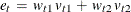, where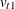and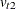are the upper and lower bounds for the tth error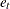, and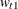and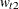represent the weight associated with the pointand. The error distribution is usually chosen to be symmetric, centered around zero, and the same across observations so that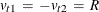, where R is the support value chosen for the problem (Golan, Judge, and Miller, 1996).

The generalized maximum entropy (GME) formulation was proposed for the ill-posed or underdetermined case where there is insufficient data to estimate the model with traditional methods.is reparameterized by defining a support for(and a set of weights in the cross entropy case), which defines a prior distribution for.

In the simplest case, each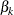is reparameterized as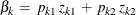, where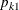and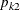represent the probabilities ranging from [0,1] for each, and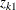and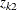represent the lower and upper bounds placed on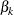. The support points,and, are usually distributed symmetrically around the most likely value forbased on some prior knowledge.

With these reparameterizations, the GME estimation problem is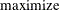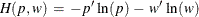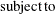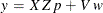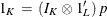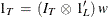where y denotes the column vector of length T of the dependent variable;denotes the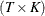matrix of observations of the independent variables; p denotes the LK column vector of weights associated with the points in Z; w denotes the LT column vector of weights associated with the points in V;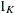,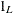, and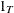are K-, L-, and T-dimensional column vectors, respectively, of ones; and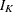and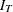are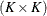and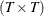dimensional identity matrices.

These equations can be rewritten using set notation as follows: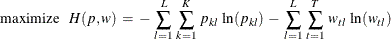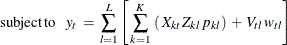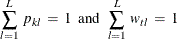The subscript l denotes the support point (l=1, 2, ..., L), k denotes the parameter (k=1, 2, ..., K), and t denotes the observation (t=1, 2, ..., T).

The GME objective is strictly concave; therefore, a unique solution exists. The optimal estimated probabilities, p and w, and the prior supports, Z and V, can be used to form the point estimates of the unknown parameters,, and the unknown errors, e.